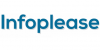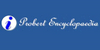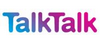### arithmetic[n] - the branch of pure mathematics dealing with the theory of numerical calculations
Found on http://www.webdictionary.co.uk/definition.php?query=arithmetic

### ArithmeticA·rith'me·tic noun [ Middle English arsmetike , Old French arismetique , Latin arithmetica , from Greek ... (sc. ...), from ... arithmetical, from ... to number, from ... number, probably from same root as English arm , the idea of counting coming from that of fitting, atta...
Found on http://www.encyclo.co.uk/webster/A/120

### arithmeticnoun the branch of pure mathematics dealing with the theory of numerical calculations
Found on https://www.encyclo.co.uk/local/20974

### Arithmetic• (n.) A book containing the principles of this science. • (n.) The science of numbers; the art of computation by figures.
Found on http://thinkexist.com/dictionary/meaning/arithmetic/

### arithmeticbranch of mathematics in which numbers, relations among numbers, and observations on numbers are studied and used to solve problems.[12 related articles]
Found on http://www.britannica.com/eb/a-z/a/100

### arithmeticA branch of mathematics concerned with doing calculations with numbers using addition, subtraction, multiplication, and division.
Found on http://www.daviddarling.info/encyclopedia/A/arithmetic.html

### arithmeticarithmetic, branch of mathematics commonly considered a separate branch but in actuality a part of algebra. Conventionally the term has been most widely applied to simple teaching of the skills of dealing with numbers for practical purposes, e.g., computation of areas, proportions, costs, and the li...
Found on http://www.infoplease.com/ce6/sci/A0804711.html

### ArithmeticArithmetic (from the Greek arithmost, number) is primarily the science of numbers. As opposed to algebra it is the practical part of the science. Although the processes of arithmetical operations are often highly complicated, they all resolve themselves into the repetition of four primary operations, addition, subtraction, multiplication, and divis...
Found on http://www.probertencyclopaedia.com/browse/GA.HTM

### arithmeticBranch of mathematics concerned with the study of numbers and their properties. The fundamental operations of arithmetic are addition, subtraction, multiplication, and division. Raising to powers (for example, squaring or cubing a number), the extraction of roots (for example, square roots), percenta...
Found on http://www.talktalk.co.uk/reference/encyclopaedia/hutchinson/m0000192.html

### ArithmeticArithmetic or arithmetics (from the Greek word ἀριθμός, arithmos `number`) is the oldest and most elementary branch of mathematics. It consists in the study of numbers, especially the properties of the traditional operations between them — addition, subtraction, multiplication and division. Arithmetic is an elementary part of number th.....
Found on http://en.wikipedia.org/wiki/Arithmetic
No exact match found